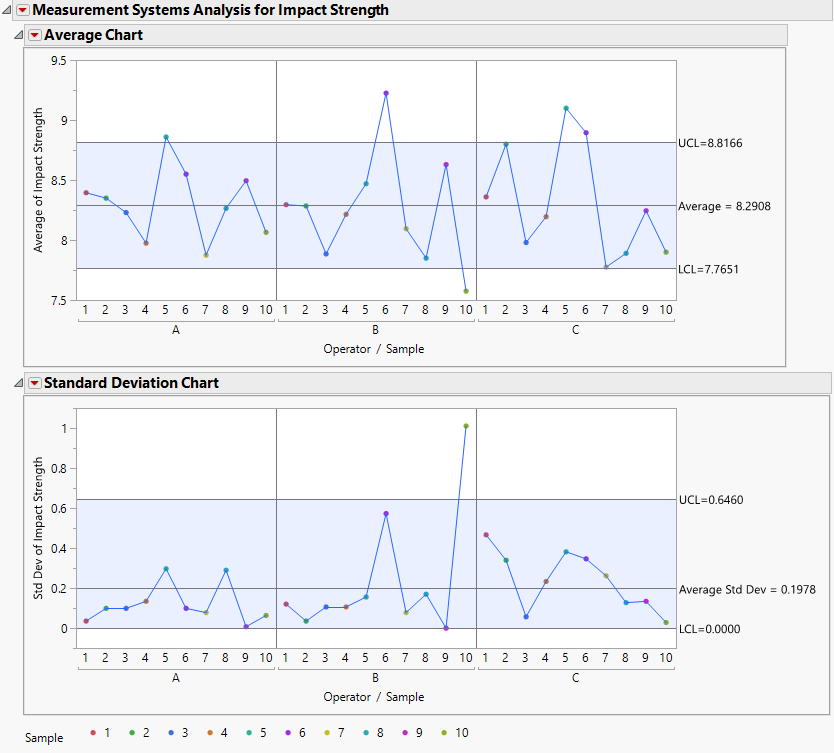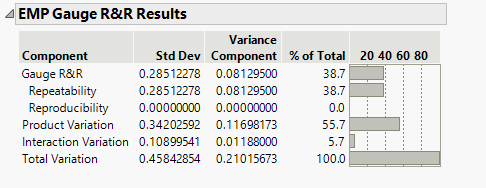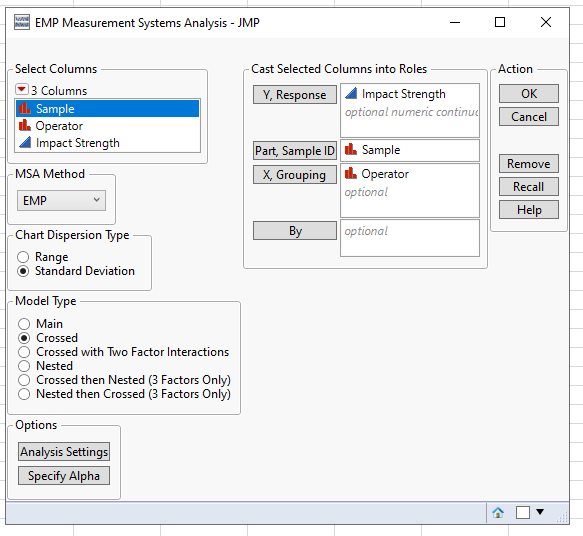Choose Language Hide Translation Bar
Highlighted

## EMP Gauge R&R - Reproducibility = 0%

Hi there!

I've ran EMP Gauge R&R and the results show the total variation coming from reproducibility is 0%. However, when I look at the data there are considerable differences in the measured variable of the same samples between operators so I can't understand how the reproducibility variation is 0%:Alicia

1 ACCEPTED SOLUTION

Accepted Solutions
Highlighted

## Re: EMP Gauge R&R - Reproducibility = 0%

First I added a column and called it measurement to represent the repeatability component of measurement precision (consecutive numbers 1-60). Then I analyzed your data using the JMP EMP (under Measurement System Analysis) and using Variability charts/control charts (attached document summarizes analysis).  To be honest, I don't know why the EMP platform gives 0% reproducibility other than the operator component is so small, perhaps it rounded it to 0? Perhaps a JMP representative can explain this? I suppose I'm old fashioned, but I do the analysis per Don Wheeler's recommendations, something I learned from him many years ago.  See Wheeler & Lyday:  "Evaluating the Measurement Process".

9 REPLIES 9
Highlighted

## Re: EMP Gauge R&R - Reproducibility = 0%

Hello Alicia
Can you please make a print screen of the input mask from the EMP platform?

"I thought about our dilemma, and I came up with a solution that I honestly think works out best for one of both of us"
Highlighted

## Re: EMP Gauge R&R - Reproducibility = 0%

Hello Mauro,

Is this what you wanted to look at?Best regards,

Alicia

Highlighted

## Re: EMP Gauge R&R - Reproducibility = 0%

You need both operator and sample in the X grouping.  Could you attach your data set?  Did you do repeated measures for each sample/operator?  How would you estimate precision repeatability without that?  In which case you will have operator and sample crossed and repeated measures nested.

Highlighted

## Re: EMP Gauge R&R - Reproducibility = 0%

Hi, please see data attached. Yes repeated measures was used - each operator A, B and C tested samples 1 - 10 twice. Thanks for your help in advance

Highlighted

## Re: EMP Gauge R&R - Reproducibility = 0%

First I added a column and called it measurement to represent the repeatability component of measurement precision (consecutive numbers 1-60). Then I analyzed your data using the JMP EMP (under Measurement System Analysis) and using Variability charts/control charts (attached document summarizes analysis).  To be honest, I don't know why the EMP platform gives 0% reproducibility other than the operator component is so small, perhaps it rounded it to 0? Perhaps a JMP representative can explain this? I suppose I'm old fashioned, but I do the analysis per Don Wheeler's recommendations, something I learned from him many years ago.  See Wheeler & Lyday:  "Evaluating the Measurement Process".

Highlighted

## Re: EMP Gauge R&R - Reproducibility = 0%

Thank you so much for the helpful replies! This makes sense to me now. Really appreciated!

Highlighted

## Re: EMP Gauge R&R - Reproducibility = 0%

The Operator variance component is negative and has therefore been set to 0.  You can see this via Fit Model.

``````Fit Model(
Y( :Impact Strength ),
Effects,
Random Effects( :Sample, :Operator, :Sample * :Operator ),
NoBounds( 1 ),
Personality( "Standard Least Squares" ),
Method( "REML" ),
Emphasis( "Minimal Report" ),
Run(
:Impact Strength << {Summary of Fit( 1 ), Analysis of Variance( 0 ), Parameter Estimates( 1 ),
Scaled Estimates( 0 ), Plot Actual by Predicted( 0 ), Plot Regression( 0 ),
Plot Residual by Predicted( 0 ), Plot Studentized Residuals( 0 ), Plot Effect Leverage( 0 ),
Plot Residual by Normal Quantiles( 0 )}
)
);``````

The reproducibility value given in the EMP Gauge R&R Results report does not include interactions and negative variance components are set to zero.

Highlighted

## Re: EMP Gauge R&R - Reproducibility = 0%

OK, that makes sense.  Negative REML...That is why JMP switched to Bayesian estimates...

Highlighted

## Re: EMP Gauge R&R - Reproducibility = 0%

Many thanks for the script... really helpful!

Article Labels

There are no labels assigned to this post.